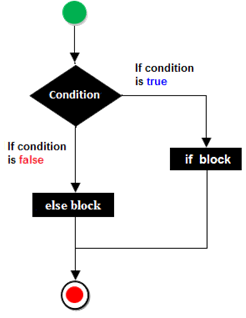If else Control Structure

The if else control structure is used where either of two action are to be performed depending upon the result of a conditional expression. It contains two block of statement. If the conditional expression evaluates to true, the statement(s) in if block are executed and if the result is false, then statement(s) in the else block get executed.

Syntax:if(conditional expression)
{
statement 1
statement 2
……………
}
else
{
statement 1
statement 2
……………
}

Example Programs

Program 

Write a program to check whether a short type number taken as input is an even number or an odd number.

Program 

Write a program to accept an int type value as a parameter and print the square of it, if it is more than 120, otherwise print its cube.

Program 

Write a program to input 5 real numbers(floating point) and find their average. If the average is greater than 50 print good, otherwise print bad.

Program 

Write a program to check whether an int type number (taken as input) is a multiple of 5 or not.

Program 

Write a program to check whether an int type number (taken as input) is a 2-digit number or not.

Program 

Write a program to check whether an int type number (taken as input) is a 3-digit number divisible by three or not.

Program 

Write a program to input 2 integers and check whether both the numbers are multiples of 7 or not.

Program 

Write a program to input three integers and check whether all of them are unique (different) from each other or not.

Program 

Write a program to input an integer and check whether it is a 5-digit number or not. If it is extract the central digit and print it.

Example

INPUT: Enter an integer: 76549

OUTPUT: Central digit: 5

INPUT: Enter an integer: 12976

OUTPUT: Central digit: 9

INPUT: Enter an integer: 126

OUTPUT: Not a 5 digit number

Program 

Write a program in Java to accept three numbers and check whether they are  Pythagorean triples or not. The program must display the message accordingly .

A "Pythagorean Triple" is a set of positive integers, a, b and c that fits the rule:

a2 + b2 = c2

Program 

Write a Java program to input a number form user and check whether number is buzz number or not.

A number is said to be Buzz Number if it ends with 7 or is divisible by 7.